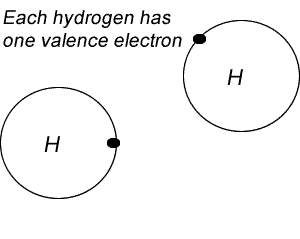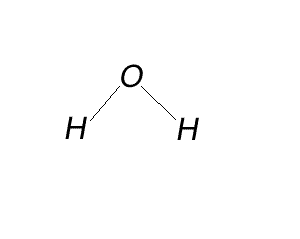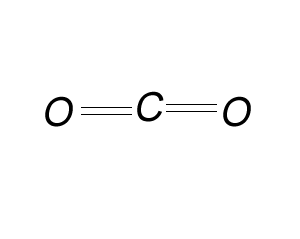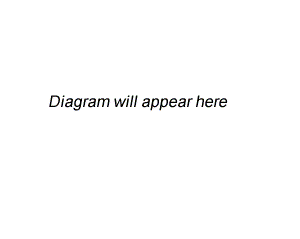Lewis dot diagrams If this is your first encounter with valence electrons and bonds, a simple analogy of bonding is given in this link. It is advisable that you read this unit first. Keep in mind that a chemical bond between two non-metals is formed by the sharing of two electrons, one from each atom. This type of bond is called a covalent bond. When two non-metal atoms react with each other they tend to share valence electrons. Valence electrons are the outer shell electrons of an atom. As a result a covalent bond is formed . Covalent bonds are very strong and form between two non-metal atoms where each bond consists of two shared electrons, one from each atom. Now, the number of covalent bonds an atom can form is called the covalency of the atom and depends on how many valence electrons short they are off eight.For example fluorine has 7 electrons and so needs only one more electron. So fluorine has a covalency of 1. Oxygen needs two electrons to achieve 8 valence electrons so its covalency is 2 Nitrogen has 5 valence electrons and so needs 3 electrons to achieve 8 valence electrons so it has a covalency of 3. Finally carbon has a covalency of 4. Guess why? An element will form as many bonds with other atoms as its covalency. For example nitrogen has a covalency of 3 so will form 3 covalent bonds with other non-metal atoms. Carbon which has a covalency of 4 will try and form 4 covalent bonds with other non-metal atoms. Of course hydrogen is an exception with 1 valence electron, it needs only one electron to fill its valence shell and so has a covalency of 1 just like all the group 7 elements. A Lewis structure or Lewis dot diagram, represents the bonds formed between two non-metal atoms as they share electrons. These diagrams show only the valence electrons of each atom as they are distributed amongst the bonded atoms. Drawing such diagrams is a great start to understanding how atoms, in particular non-metal atoms, combine to form molecules and the resulting shape of the molecule. Non-metal atoms bond by sharing valence or outer shell electrons with each other, this often results in the formation of molecules. Non-metal as well as metal atoms, with the exception of hydrogen, will interact with other atoms so as to gain 8 valence electrons. This is known as the Octet Rule. Hydrogen is the exception as it only needs two valence electrons to achieve a low energy state.   Lets start with carbon dioxide. On the right is a Lewis dot diagram representing the simplest molecule in nature, hydrogen. To draw Lewis dot diagrams follow the steps below. 1) Draw each atom with its valence electrons only. 2) Overlap the outer shell of each atom so that electrons overlap two atoms. 3) The number of electrons that overlap belong to both atoms and are counted when we obey the Octet Rule, with the obvious exception of hydrogen. 4) Place all electrons in pairs. The pair of electrons shared are called bonding pairs and the ones that are not shared are called non-bonding pairs, or lone pairs. 5) Replace each pair with a line. A line that goes between two atoms represents a bonding pair and is known as a covalent bond. We can have a single bond, double bond or triple bond as the most common types of bonds. The number of bonding pairs for each atom should be equal to the covalency of that atom.Try the steps for H2O 1) Draw each atom with its valence electrons only. 2) Overlap the outer shell of each atom so that electrons overlap two atoms. 3) The number of electrons that overlap belong to both atoms and are counted when we obey the Octet Rule, with the obvious exception of hydrogen. 4) Place all electrons in pairs. The pair of electrons shared are called bonding pairs and the ones that are not shared are called non-bonding pairs, or lone pairs. 5) Replace each pair with a line. Each line represents a bonding pair if it goes between two atoms and is known as a covalent bond. The number of bonding pairs for each atom should be equal to the covalency of that atom.Try the same steps for CO2 1) Draw each atom with its valence electrons only. 2) Overlap the outer shell of each atom so that electrons overlap two atoms. 3) The number of electrons that overlap belong to both atoms and are counted when we obey the Octet Rule, with the obvious exception of hydrogen. 4) Place all electrons in pairs. The pair of electrons shared are called bonding pairs and the ones that are not shared are called non-bonding pairs, or lone pairs. The number of bonding pairs for each atom should be equal to the covalency of that atom. 5) Replace each pair with a line. Each line represents a bonding pair if it goes between two atoms and is known as a covalent bond. The number of bonding pairs for each atom should be equal to the covalency of that atom.View the video on the right to see an animation of the process mentioned above of how to draw a Lewis dot diagram of CO2. Its easy to draw Lewis dot diagrams when we know the molecular formula but can we produce Lewis dot diagrams when we do not know the formula of a compound. Lets do one for the compound formed when phosphorus and bromine react. Write the formula of the compound formed. Using the covalency of the atom this is pretty straight forward. Before we start drawing Lewis dot diagrams we must first work out the formula of the compound formed: a) Write the element and its covalency as a subscript. b) Swap the subscripts and divide by the smallest number only if the larger number is divisible by the smaller number. c) Write the formula 1) Draw each atom with its valence electrons only. 2) Overlap the outer shell of each atom so that electrons overlap two atoms. 3) The number of electrons that overlap belong to both atoms and are counted when we obey the Octet Rule, with the obvious exception of hydrogen. 4) Place all electrons in pairs. The pair of electrons shared are called bonding pairs and the ones that are not shared are called non-bonding pairs, or lone pairs. 5) Replace each pair with a line. Each line represents a bonding pair if it goes between two atoms and is known as a covalent bond. The number of bonding pairs for each atom should be equal to the covalency of that atom.Draw a Lewis dot structure for the molecule formed when silicon and oxygen combine. a) Write the elements and their covalency as a subscript. b) Swap the subscripts and divide by the smallest number. c) Write the formula Follow the steps listed above to draw the Lewis dot structure.1) Draw the Lewis dot structures for: a) H3COH b) C2H2 c) N2 d) N2H2 . e) CH4 f) HCOOH Solutions Stick structures After looking at the stick structures of the following molecules click to see their three dimensional shapes. a) CH4 Click to see the 3D image of the molecular shape. b) H3COH Click to see the 3D image of the molecular shape. c) N2H2 Click to see the 3D image of the molecular shape2) Which of these pair of atoms will form covalent bonds with each other? Aluminium and boron nitrogen and lithium nitrogen and oxygen oxygen and iron 3) How many covalent bonds can the following atoms form? a) Nitrogen 3 4 5 1 0 2 b) Na 3 4 5 1 0 2 c) Oxygen 3 4 5 1 0 2 d) Chlorine 3 4 5 1 0 2 e) Phosphorus 3 4 5 1 0 2 f) Sulfur 3 4 5 1 0 2 4) Which is the central atom in the following molecules?: a) CH4, C H N O b) CO2, C H N O c) NH3 C H N O 5) How many covalent bonds are present the following molecules? a) CH4, 3 4 5 1 0 2 b) CO2, 3 4 5 1 0 2 c) NH3 3 4 5 1 0 2 Continue with covalency and drawing molecules